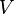# Volume facts for kids

Kids Encyclopedia Facts

The volume of an object is a measure of the amount of space occupied by that object, and is not to be confused with mass. The volume of a mountain is much larger than the volume of a rock, for instance.

By convention, the word volume implies a three-dimensional context where:

• The length is the longest distance between the object's extremities.
• The width (or breadth) refers to the size of the object in a direction perpendicular to its length.
• The height (or depth) stands for the size of that object in the direction perpendicular to both the length and the width.

For objects at or near the Earth's surface, height or depth often refers to the dimension of the object along the local vertical. All physical objects occupy a volume, even if some are so thin that they appear to be two-dimensional, like a sheet of paper.

## Units of volume

The unit of volume in the International System of Units is the cubic meter, which is represented by the symbol m3.

In some fields or applications, one often uses different units to simplify the discussions or writings. For instance:

• Everyday quantities of liquids are often measured in units of a litre, written as L, which is the volume occupied by a cubic decimetre.
• Large quantities of liquids, such as oil, and sometimes other materials, may be measured and traded in units of barrels. There are many different reference volumes called barrels, depending on the nature of the content.

Traditional units are still in encountered in some countries: Imperial units such as the gallon or the fluid ounce were in widespread use within the British Empire. Some of them are still popular in the United States, which also uses units like the bushel, the quart, the cup and the teaspoon (in cooking recipes, for example). See U.S. customary units for more examples.

## Non-conservation of volume

The volume of an object is not a fundamental property of that object: it can change with environmental conditions such as pressure and temperature, especially if the object is highly compressible.

The volume of a mixture of fluids (liquids, gases) may or may not be equal to the sum of their volumes before they were mixed.

## Measuring a volume

In mathematics, the volume of simple geometric objects, written$V$, can often be calculated on the basis of their shape and dimensions:

• The volume of a perfect cube of side c is c3.
• The volume of a rectangular box is the product of its three linear dimensions: length, width and length.
• The volume of a parallelepiped of sides a, b and c is a × b × c.
• The volume of a sphere of radius r is (4/3) π r3.

The volume of a gas is typically that of its container, but it could be ill-defined, as in the case of the atmosphere, which has no clear upper limit. The volume of a liquid is often measured by pouring it into a graduated container. The volume of a small solid can be estimated by immersing it into a graduated container partially filled with a known amount of liquid, provided the solid is not soluble in the liquid.

## Related pagesVolume Facts for Kids. Kiddle Encyclopedia.